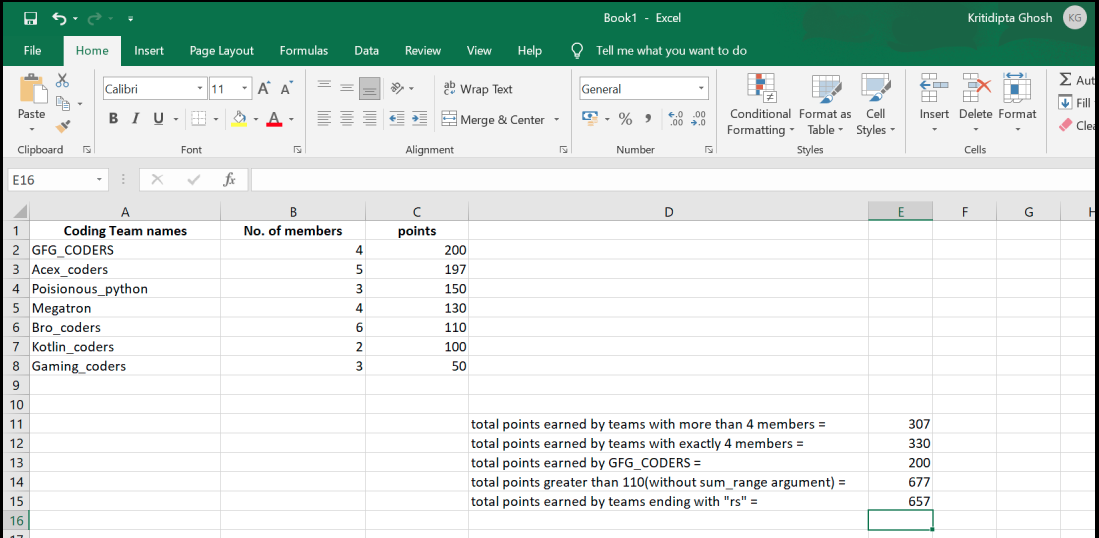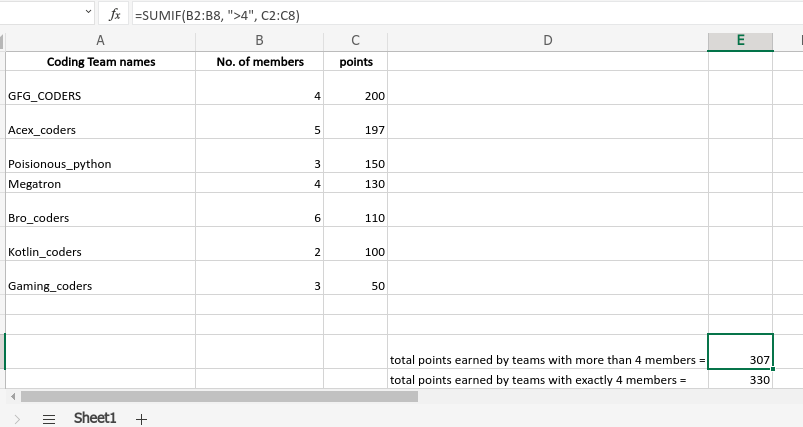# SUMIF Function in Excel with Examples

• Difficulty Level : Easy
• Last Updated : 27 Apr, 2021

Excel is a great tool to use in data collection and entry and nowadays it is widely used worldwide. To make the tasks of data management easier Excel has some built-in functions and formulas. SUMIF is such a function. This function easily sums the particular numbers in a large dataset. This is basically a worksheet function and comes under the Math & Trigonometry function

This function does exactly what its name indicates. Using this function we can get the ‘SUM’ of numbers of a particular range ‘IF’ the given criteria is satisfied. This function actually evaluates the sum of a particular range applying a condition. And in this function, we can apply criteria to one range and sum up another range according to the criteria.

Syntax:

SUMIF(range, criteria, [sum_range](optional))

***[sum_range] is an optional argument.

This function accepts range, criteria(condition), and sum_range(optional argument) as its argument and returns the sum of the sum_range or the range according to the given arguments. The arguments are discussed below.

Arguments:

Arguments of the SUMIF function are the following:

• range(Required): This is the range of the cells that will be evaluated to check the given criteria or condition. These cells may contain numbers or names, dates(must be in standard excel formats), arrays, references(that contains numbers).
• criteria(Required): This argument is the criteria that need to be satisfied to add a cell of the given range. It can be a number, expression(logical or mathematical), text, reference, or a function. If the criteria is a mathematical or logical expression it must be written within double quote(Eg: ” >1000″). On the other hand, if the criteria is a number then the double quote is not required(Eg: 56). There are some special symbols like a question mark(‘?’) And asterisk(‘*’) with some special use:
1.  A question mark(?) can be used to match a single character.
2. An asterisk(*) can be used to match a sequence of characters.
•   And if we want to match a question mark(?) or an asterisk in the cell we need to use a tilde sign before them(Eg: “~?”).
• sum_range(Optional): This is the actual range that will be added cell by cell if the given criteria meet. But if this argument is not provided then the SUMIF function will return the sum of the range argument. sum_range should be of the same dimension of range argument for better performance.

Return Value:

This function sums the numbers in the given range and returns the numerical value of the sum.

Example:

An Excel sheet has been taken as an example and the SUMIF function has been used in several formats.

Then we will apply the SUMIF() function to the above table:

Output:Output Excel SheetUsing SUMIF function.

My Personal Notes arrow_drop_up